# RS Aggarwal Class 7 Solutions Chapter 10 Percentage Ex 10C

In this chapter, we provide RS Aggarwal Solutions for Class 7 Chapter 10 Percentage Ex 10C for English medium students, Which will very helpful for every student in their exams. Students can download the latest RS Aggarwal Solutions for Class 7 Chapter 10 Percentage Ex 10C Maths pdf, free RS Aggarwal Solutions Class 7 Chapter 10 Percentage Ex 10C Maths book pdf download. Now you will get step by step solution to each question.

### RS Aggarwal Solutions for Class 7 Chapter 10 Percentage Ex 10C Download PDF

Question 1.
Solution:
(b) 34 = 34 x 100 = 75 %

Question 2.
Solution:
(c)
2 : 5 = 25 = 25 x 100 = 40%

Question 3.
Solution:
(c)Question 4.
Solution:
(c) x% of 75 = 9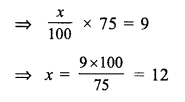Question 5.
Solution:
(d)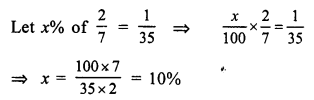Question 6.
Solution:
(b)
Let x% of 1 day = 36 minutes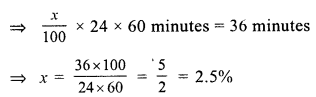Question 7.
Solution:
(a)
Let x be the required numberQuestion 8.
Solution:
(b)
Let x be the required number, thenQuestion 9.
Solution:
(d)
Let ore = x, then
5% of x = 400g
xx5100 400Question 10.
Solution:
(b)
Let gross value of T.V = x
Commission = 10%
After deducting commission, the value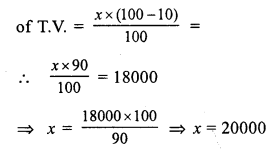Question 11.
Solution:
(b)
Increase in salary = 25%
Let original salary = xQuestion 12.
Solution:
(c)
Let x be the number of total examinees
No. of examinees passedQuestion 13.
Solution:
(c)
Let total number of apples = xQuestion 14.
Solution:
(b)
Present value of machine = Rs. 25000
Rate of depreciation = 10% p.a.
Value of machine after one yearQuestion 15.
Solution:
(c) Let x be numbers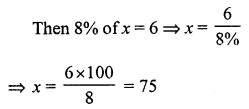Question 16.
Solution:
(c) 60% of 450
= 60100 x 450 = 270

Question 17.
Solution:
(d) Rate of reduction = 6%
Price after reduction = Rs. 658Question 18.
Solution:
(b) Boys = 70% of students
No. of girls = 240
Girls percentage = 100 – 70 = 30%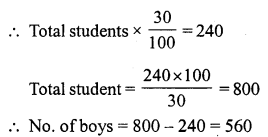Question 19.
Solution:
(c) Let number = x
11% of x – 7% of x = 18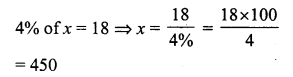Question 20.
Solution:
(a) Let number = x
35% of x + 39 = xQuestion 21.
Solution:
(c) Pass marks = 36%
A students get =145 marks
But failed by 3 5 marks
Then pass marks = 145 + 35 = 180
Maximum marks = 180×10036 = 500

Question 22.
Solution:
(d) Let number be = x
Then decreasing by 40%All Chapter RS Aggarwal Solutions For Class 7 Maths

—————————————————————————–

All Subject NCERT Exemplar Problems Solutions For Class 7

All Subject NCERT Solutions For Class 7

*************************************************

I think you got complete solutions for this chapter. If You have any queries regarding this chapter, please comment on the below section our subject teacher will answer you. We tried our best to give complete solutions so you got good marks in your exam.

If these solutions have helped you, you can also share rsaggarwalsolutions.in to your friends.